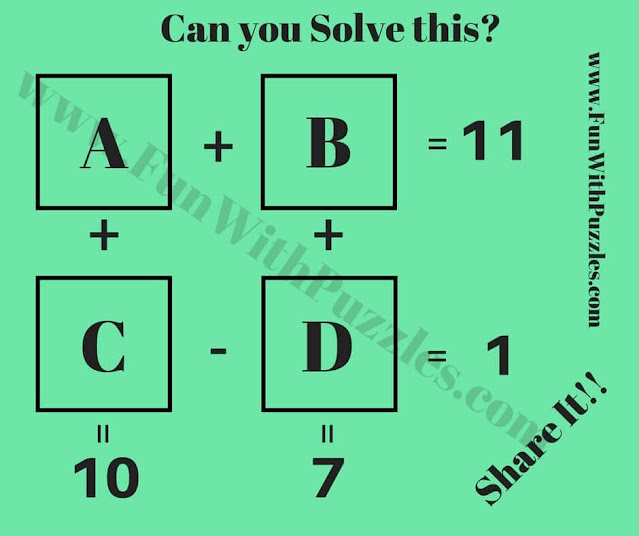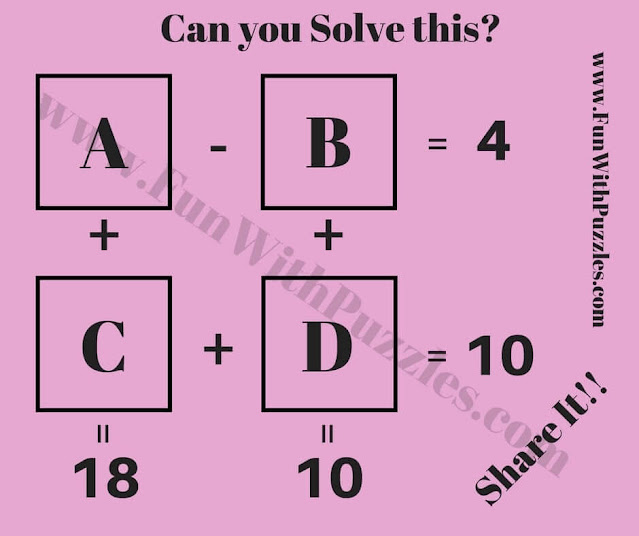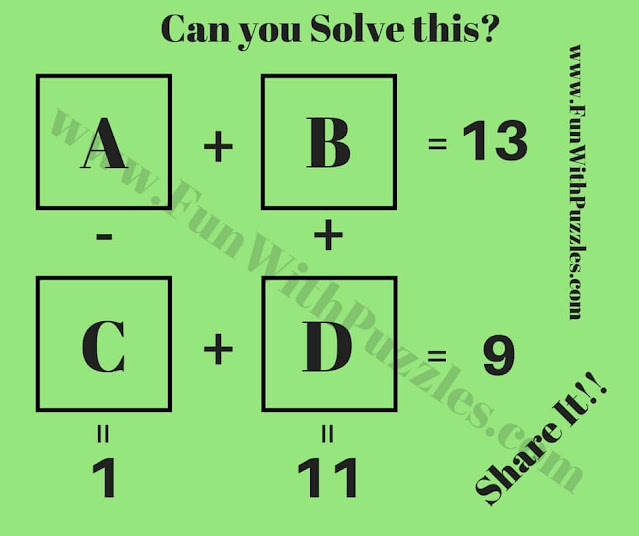Mathematics is a very interesting subject. Mathematics can be taught in very innovative and interesting ways. There are many different types of Maths Puzzles and Math Brain Teasers that can be created. Here we are presenting a very interesting way in which Mathematical equations are created. All these Maths Brain Teasers are printable and can be used in schools as worksheets.
In these Maths Puzzles Brain Teasers, there are four variables represented by A, B, C, and D. There are four equations as represented in each of the Math Puzzle Worksheets. Your challenge is to solve these equations and find values of A, B, C, and D in each of these Maths Brain Teasers.
Answers to each of these Math Brain Teaser puzzles are given at the end.1. Can you solve this to find the values of A, B, C, and D?2. Can you find which numbers will replace A, B, C, and D?3. Solve it to find values of A, B, C, and D.4. Solve these equations to find A, B, C, and D.5. Can you quickly solve these to find A, B, C, and D?

Try out some interesting Fun Puzzles and Brain Teasers as mentioned below

## List of more Puzzles and Brain Teasers

1. Cool Maths Brain Teasers with Answers: It contains many different types of Maths Brain Teasers, which are logical and mathematical.

2. Crack the Code to Open the Lock: It contains five Mind Logical challenges to crack the code for opening the lock.

3. Quick Solving Maths Puzzles for Kids: These are Quick Maths Brain Teasers for Kids.

4. What Comes Next Puzzles: It contains the Maths Puzzles in which your challenge is to find the pattern in the given numbers in the series and then find the next number in the series.

Answers to these Maths Puzzles Brain Teasers are given below. Try to solve these Maths puzzles by yourself and use them to check your answers' correctness.
1)
A= 6.5
B= 4.5
C= 3.5
D= 2.5

2)
A= 6
B= 7
C= 3
D= 1

3)
A=11
B=7
C=7
D=3

4)
A=6
B=7
C=5
D=4

5)
A=12
B=1
C=5
D=3